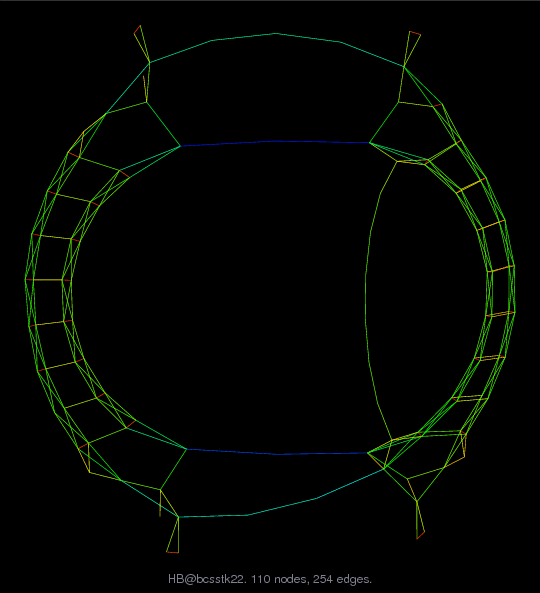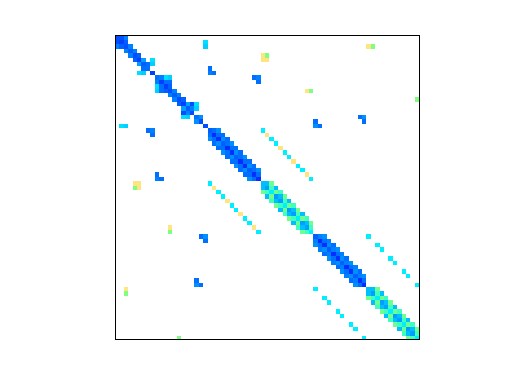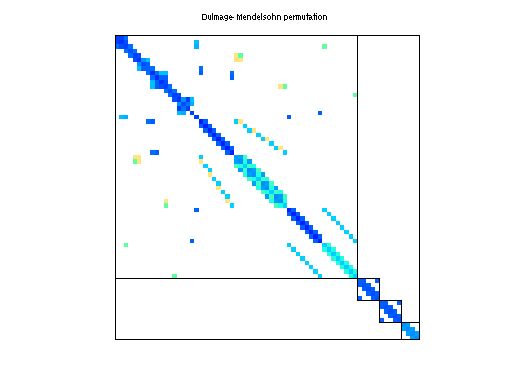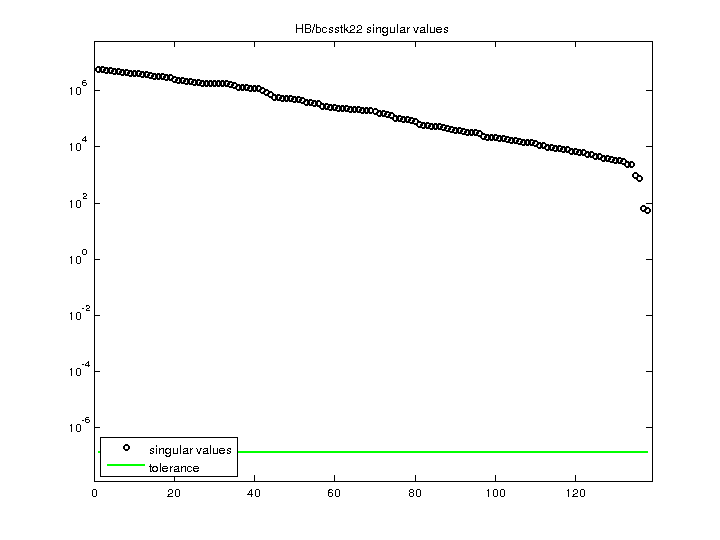Matrix: HB/bcsstk22

Description: SYMMETRIC STIFFNESS MATRIX - TEXTILE LOOM FRAME(undirected graph drawing)• Matrix group: HB
• download as a MATLAB mat-file, file size: 3 KB. Use UFget(44) or UFget('HB/bcsstk22') in MATLAB.

 Matrix properties number of rows 138 number of columns 138 nonzeros 696 structural full rank? yes structural rank 138 # of blocks from dmperm 4 # strongly connected comp. 4 explicit zero entries 0 nonzero pattern symmetry symmetric numeric value symmetry symmetric type real structure symmetric Cholesky candidate? yes positive definite? yes

 author J. Lewis editor I. Duff, R. Grimes, J. Lewis date 1984 kind structural problem 2D/3D problem? yes

 Ordering statistics: result nnz(chol(P*(A+A'+s*I)*P')) with AMD 680 Cholesky flop count 4.2e+03 nnz(L+U), no partial pivoting, with AMD 1,222 nnz(V) for QR, upper bound nnz(L) for LU, with COLAMD 791 nnz(R) for QR, upper bound nnz(U) for LU, with COLAMD 1,398

 SVD-based statistics: norm(A) 5.85012e+06 min(svd(A)) 52.8387 cond(A) 110717 rank(A) 138 sprank(A)-rank(A) 0 null space dimension 0 full numerical rank? yes

 singular values (MAT file): click here SVD method used: s = svd (full (A)) ; status: ok